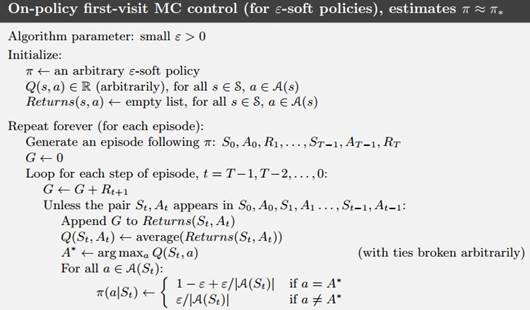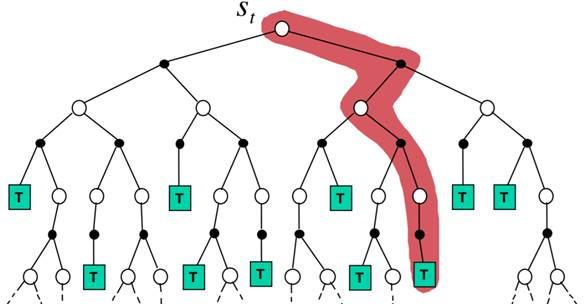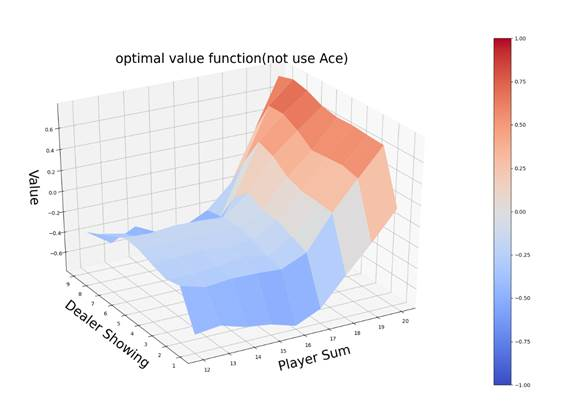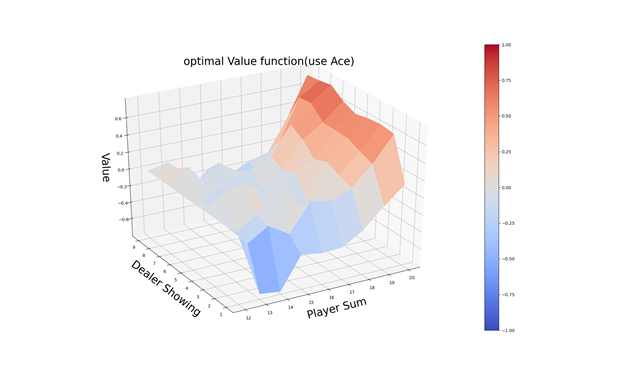## 三、实验过程

import gym
import numpy as np
from collections import defaultdict
import matplotlib
import matplotlib.pyplot as plt

env = gym.make('Blackjack-v0')
observation = env.reset()
print(env.action_space, env.observation_space, sep='\n')def make_epsilon_greedy_policy(Q_table, nA, epsilon):
def generate_policy(observation):
prob_A = np.ones(nA) * epsilon / nA
optimal_a = np.argmax(Q_table[observation])
prob_A[optimal_a] += (1.0 - epsilon)
return prob_A

return generate_policy

MC算法是逐幕进行的，所以我们要根据策略来生成一幕数据。

def MC_control(env, iteration_times=500000, epsilon=0.1, discount_factor=1.0):
Return, Count, Q_table = defaultdict(float), defaultdict(float), defaultdict(lambda: np.zeros(env.action_space.n))
policy = make_epsilon_greedy_policy(Q_table, env.action_space.n, epsilon)
for i in range(iteration_times):
if i % 1000 == 0:
print(str(i) + "次")

trajectory = generate_one_episode(env, policy)
s_a_pairs = set([(x, x) for x in trajectory])
for state, action in s_a_pairs:
s_a = (state, action)
first_visit_id = next(i for i, x in enumerate(trajectory) if x == state and x == action)
G = sum([x * (discount_factor ** i) for i, x in enumerate(trajectory[first_visit_id:])])
Return[s_a] += G
Count[s_a] += 1.
Q_table[state][action] = Return[s_a] / Count[s_a]
return policy, Q_table

def plot_value_function(Q_table):
x = np.arange(12, 21)
y = np.arange(1, 10)
X, Y = np.meshgrid(x, y)
Z_noace = np.apply_along_axis(lambda x: Q_table[(x, x, False)], 2, np.dstack([X, Y]))
Z_ace = np.apply_along_axis(lambda x: Q_table[(x, x, True)], 2, np.dstack([X, Y]))
def plot_surface(X, Y, Z, title):
代码过长略

## 四、实验结果